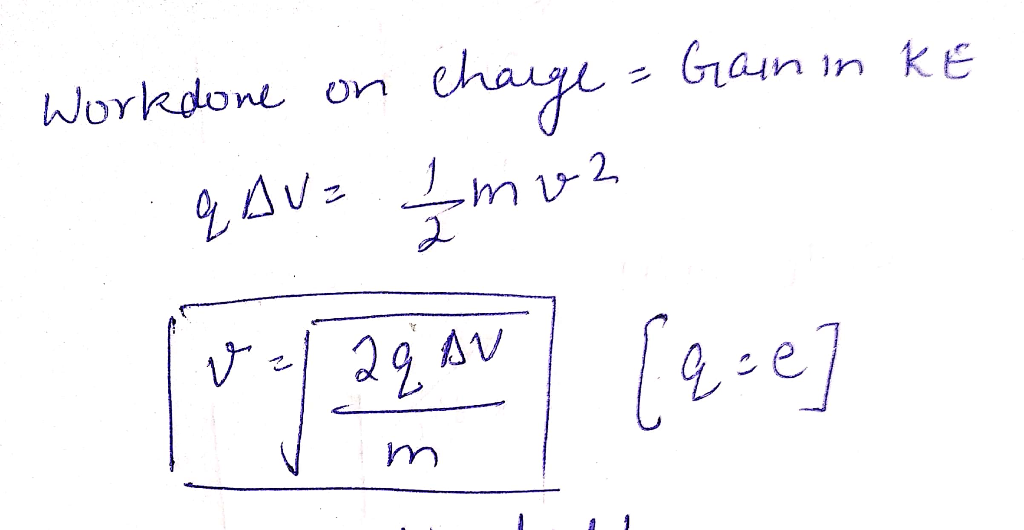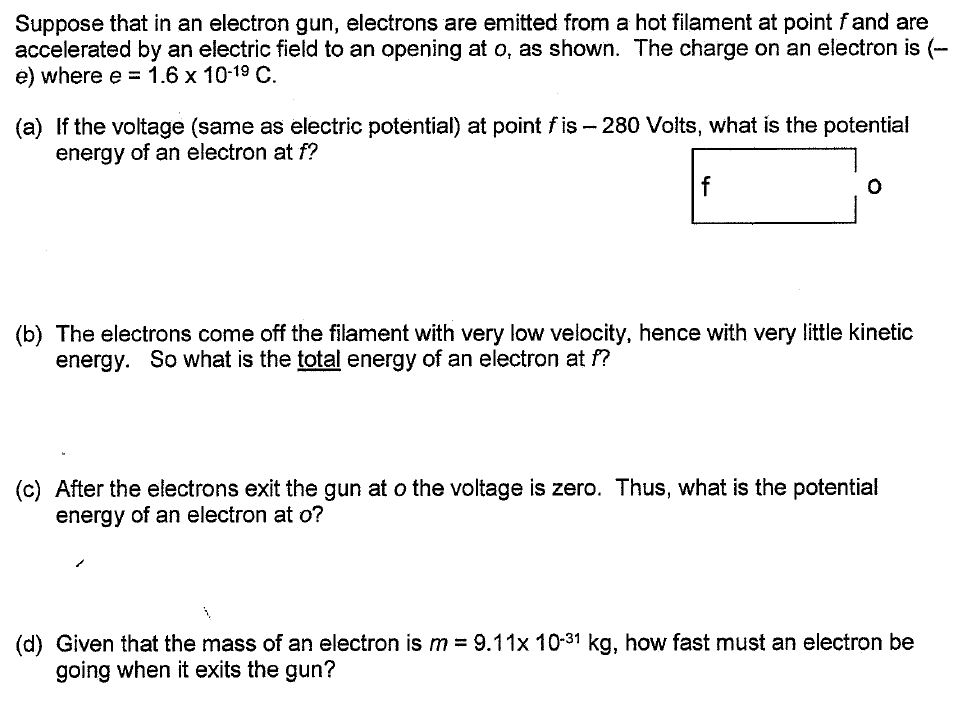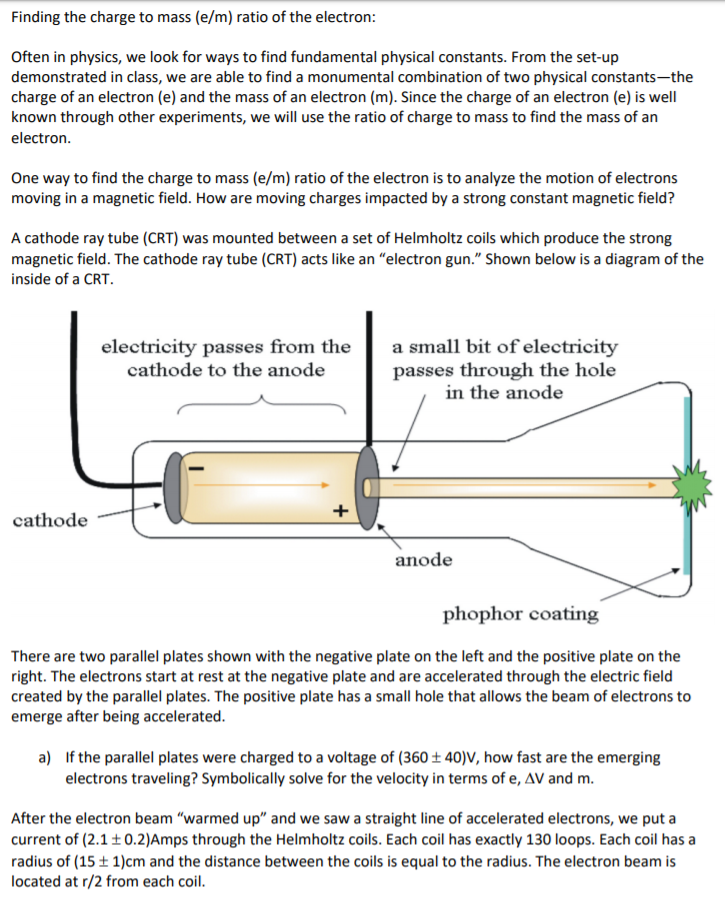Question

# An electron is “boiled” from a filament. This means that the electron, with charge e and...

An electron is “boiled” from a filament. This means that the electron, with charge e and mass m, starts from rest near the filament. It is then accelerated by a potential difference of ∆V volts. What is the speed v of the electron? Solve symbolically and put your work here:#### Earn Coins

Coins can be redeemed for fabulous gifts.

Similar Homework Help Questions
• ### Suppose that in an electron gun, electrons are emitted from a hot filament at point f...Suppose that in an electron gun, electrons are emitted from a hot filament at point f and are accelerated by an electric field to an opening at o, as shown. The charge on an electron is (-e) where e = 1.6 times 10^-19 C. (a) If the voltage (same as electric potential) at point f is - 280 Volts, what is the potential energy of an electron at f? (b) The electrons come off the filament with very low velocity,...

• ### an electron and a proton (electron with charge -e, and proton with charge +e) are accelerated...

an electron and a proton (electron with charge -e, and proton with charge +e) are accelerated through a potential of 100 volts. after this acceleration we can say that: a) the mass of the electron and the mass of the proton must be the same b) the speed of the electron and the proton are the same, and their kinetic energies are the same c) the momentum of the electron and the proton are the same e) the spin of...

• ### Finding the charge to mass (e/m) ratio of the electron Often in physics, we look for ways to find fundamental physical...Finding the charge to mass (e/m) ratio of the electron Often in physics, we look for ways to find fundamental physical constants. From the set-up demonstrated in class, we are able to find a monumental combination of two physical constants-the charge of an electron (e) and the mass of an electron (m). Since the charge of an electron (e) is well known through other experiments, we will use the ratio of charge to mass to find the mass of an...

• ### Finding the charge to mass (e/m) ratio of the electron Often in physics, we look for ways to find fundamental physical...Finding the charge to mass (e/m) ratio of the electron Often in physics, we look for ways to find fundamental physical constants. From the set-up demonstrated in class, we are able to find a monumental combination of two physical constants-the charge of an electron (e) and the mass of an electron (m). Since the charge of an electron (e) is well known through other experiments, we will use the ratio of charge to mass to find the mass of an...

• ### Electrons (mass m, charge –e) are accelerated from rest through a potential difference V and are...

Electrons (mass m, charge –e) are accelerated from rest through a potential difference V and are then deflected by a magnetic field B that is perpendicular to their velocity. The radius of the resulting electron trajectory is:

• ### An electron (mass m = 9.11 x 10-31 kg, charge e = 1.6 x 10-19 C)...

An electron (mass m = 9.11 x 10-31 kg, charge e = 1.6 x 10-19 C) are accelerated from rest through a potential difference V = 450 V and are then deflected by a magnetic field (B = 0.4 T) that is perpendicular to their velocity. The radius of the resulting electron trajectory is:

• ### An electron has been accelerated from rest through a potential difference of 700 V . A)What...

An electron has been accelerated from rest through a potential difference of 700 V . A)What is the electron's kinetic energy, in electron volts? B)What is the electron's speed?

• ### An electron is accelerated from rest through a potential difference that has a magnitude of 2.70...

An electron is accelerated from rest through a potential difference that has a magnitude of 2.70 × 107 V. The mass of the electron is 9.11 × 10-31 kg, and the negative charge of the electron has a magnitude of 1.60 × 10-19 C. (a) What is the relativistic kinetic energy (in joules) of the electron? (b) What is the speed of the electron? Express your answer as a multiple of c, the speed of light in a vacuum.

• ### An electron has been accelerated from rest through a potential difference of 700 V . What...

An electron has been accelerated from rest through a potential difference of 700 V . What is the electron's kinetic energy, in electron volts? What is the electron's kinetic energy, in joules? What is the electron's speed?

• ### What is the final speed of a free electron accelerated from rest through a potential difference...

What is the final speed of a free electron accelerated from rest through a potential difference of -100 V? The mass of the electron is 9.11x10-31kg. You need to express the speed in km/s.